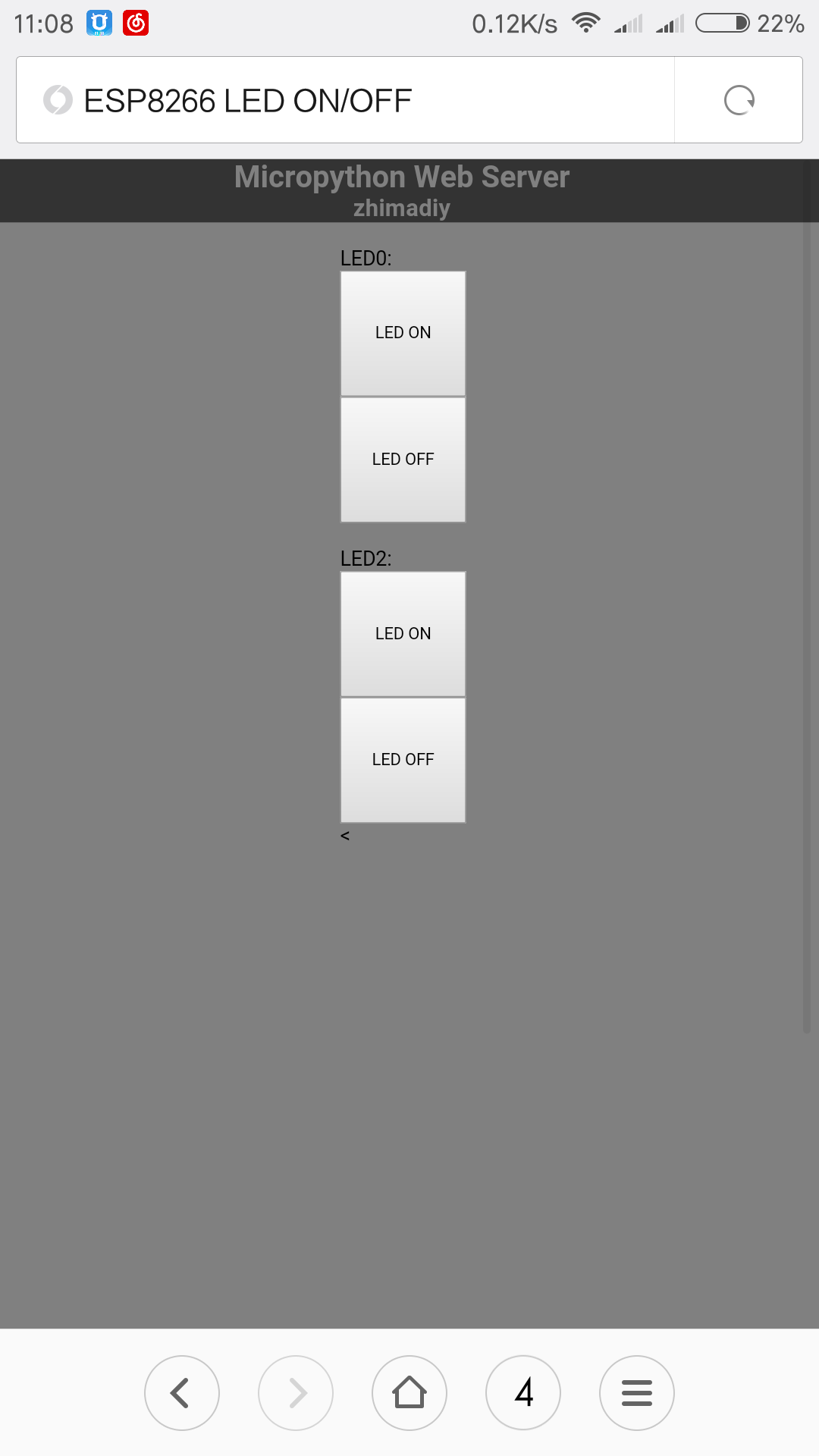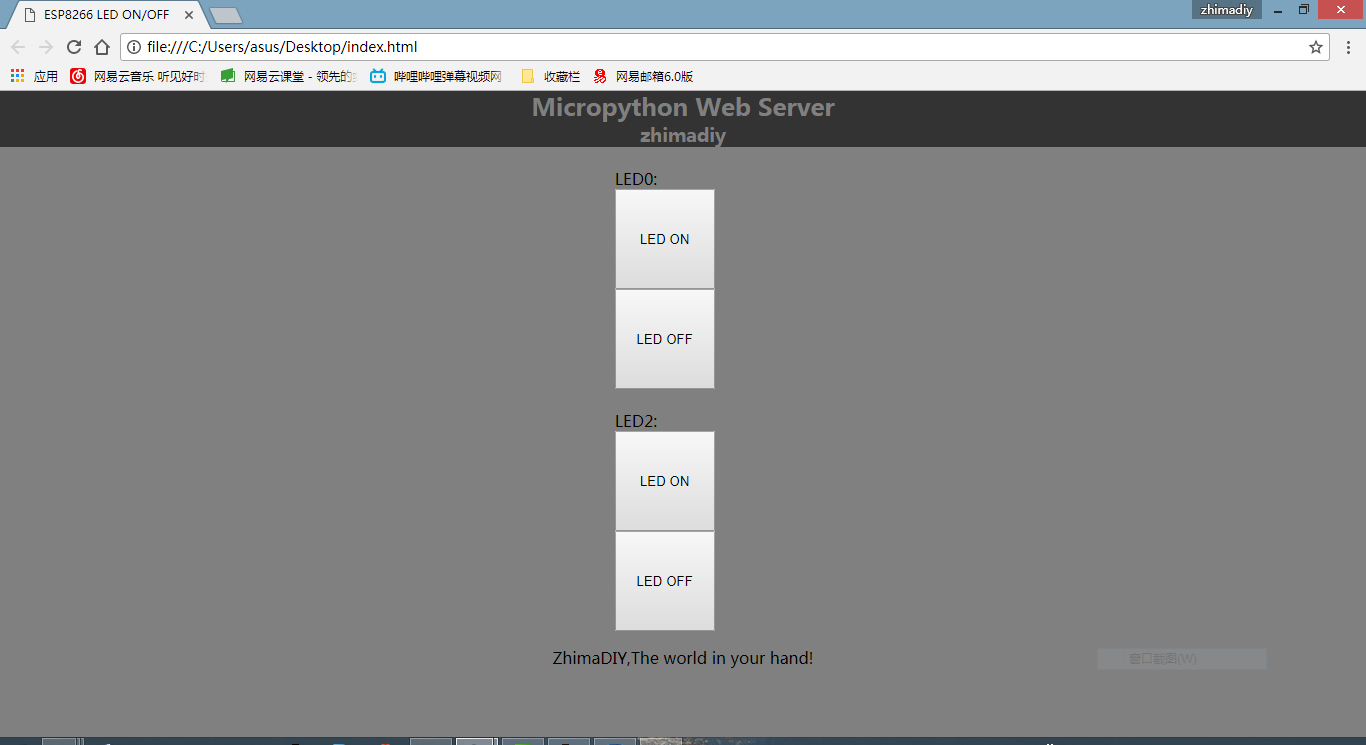# 用开发板搭建一个简单的Web服务器

Python提供了访问底层操作系统Socket接口的全部方法，需要的时候这些接口可以为你提供灵活而强有力的功能。

—-John Goerzen《Python网络编程基础》``` import network ap = network.WLAN(network.AP_IF) #设为AP模式 ap.active(True) ap.config(essid='ZhimaDIY',password="12345678") #配置wifi接入点 ```

``` import socket import machine import os```

#Setup PINS
LED0 = machine.Pin(16, machine.Pin.OUT)
LED2 = machine.Pin(2, machine.Pin.OUT)

#Setup Socket WebServer
s = socket.socket(socket.AF_INET, socket.SOCK_STREAM)
s.bind((”, 80))
s.listen(5)
page=open(‘index.html’)
page.close()
while True:
print(“Got a connection from %s” % str(addr))
request = conn.recv(1024)
print(“Content = %s” % str(request))
request = str(request)
LEDON0 = request.find(‘/?LED=ON0’)
LEDOFF0 = request.find(‘/?LED=OFF0’)
LEDON2 = request.find(‘/?LED=ON2’)
LEDOFF2 = request.find(‘/?LED=OFF2’)
#print(“Data: ” + str(LEDON0))
#print(“Data2: ” + str(LEDOFF0))
if LEDON0 == 6:
print(‘TURN LED0 ON’)
LED0.value(0)
if LEDOFF0 == 6:
print(‘TURN LED0 OFF’)
LED0.value(1)
if LEDON2 == 6:
print(‘TURN LED2 ON’)
LED2.value(0)
if LEDOFF2 == 6:
print(‘TURN LED2 OFF’)
LED2.value(1)
response = html
conn.send(response)
conn.close()
s.close()

Index.html是网页的主体部分，这个网页可能有点丑，大家可以通过修改html和css使网页更优美。